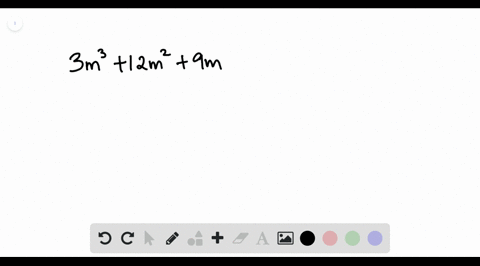Enroll in one of our FREE online STEM summer camps. Space is limited so join now!View Summer Courses### Factor each polynomial. If a polynomial cannot be…

01:00Other Schools

Need more help? Fill out this quick form to get professional live tutoring.

Get live tutoring
Problem 16

Factor each polynomial. If a polynomial cannot be factored, write prime. Factor out the greatest common factor as necessary.
$$6 a^{2}-48 a-120$$

$6 a^{2}-48 a-120=6(a-10)(a+2)$

## Discussion

You must be signed in to discuss.

## Video Transcript

we're asked to factor those trying a little here at first glance and looks like it's a trying a meal where the leading coefficient is not one. But if you look closely, all these three cool, efficient are multiples of six, which means we can actually factor out of six from all three turns our left with a squared minus eight a minus 20. So now this train a meal here thus have a leading coefficient of one, and we know how to factor that. All we need to do is find two numbers that multiply together to be negative 20 and add together to be negative eight. So that would be negative. 10 and positive, too.